This document provides a brief introduction to Global Sensitivity Analysis (GSA) and a workflow to perform GSA in R using the SAFE (Sensitivity Analysis For Everybody) toolbox (References 1-2), using as an example an early development version of JBA’s Global Flood Model (Reference 3). This is a proof of concept only, and the results shown should not be considered exemplificative of JBA’s Global Flood Model version for release.

The Global Flood Model is run for Thailand and we test the influence of variations in some of its inputs on the uncertainty of the outputs (i.e. average annual loss and losses for various return periods).

We consider both the case when the model is run in R and when it is run in a different environment (e.g. Python, as in this case), in the latter case we guide the user through the steps to upload the simulated model inputs and outputs through csv files.

But first,

## What is Sensitivity Analysis? and why shall we use it?

Sensitivity Analysis (SA) is:

a set of mathematical techniques which investigate how uncertainty in the output of a numerical model can be attributed to variations of its input factors.

Benefits:

1. Better understanding of the model

Evaluation of model behaviour beyond default set-up

2. “Sanity check” of the model

Does the model meet the expectations (model validation)?

3. Prioritize investments for uncertainty reduction

Identify sensitive inputs for computer-intensive calibration, acquisition of new data, etc.

4. More transparent and robust decisions

Understand main impacts of uncertainty on modelling outcome and thus on decisions

## How Global Sensitivity Analysis (GSA) works

Let’s say we want to test how the uncertainty of the selected model input factors influences the variability of the model output.

An input factor is any element that can be changed before running the model. In general, input factors could be equations implemented in the model, set-up choices needed for the model execution on a computer, parameters and input data. Input factors could be both continuous and discrete variables.

The output can be any variable that is obtained after the model’s execution.

Before executing the model, we will simulate the inputs in their range of variability and then run the model so that for each simulation all the 3 inputs vary simultaneously (Input Sampling step). For every output of interest a probability distribution is obtained, after which sensitivity analysis with the GSA method of choice is performed, which allows to obtain a set of sensitivity indices for each output (i.e. one per input, which shows the relative influence input factors have on the output) (Reference 4,6).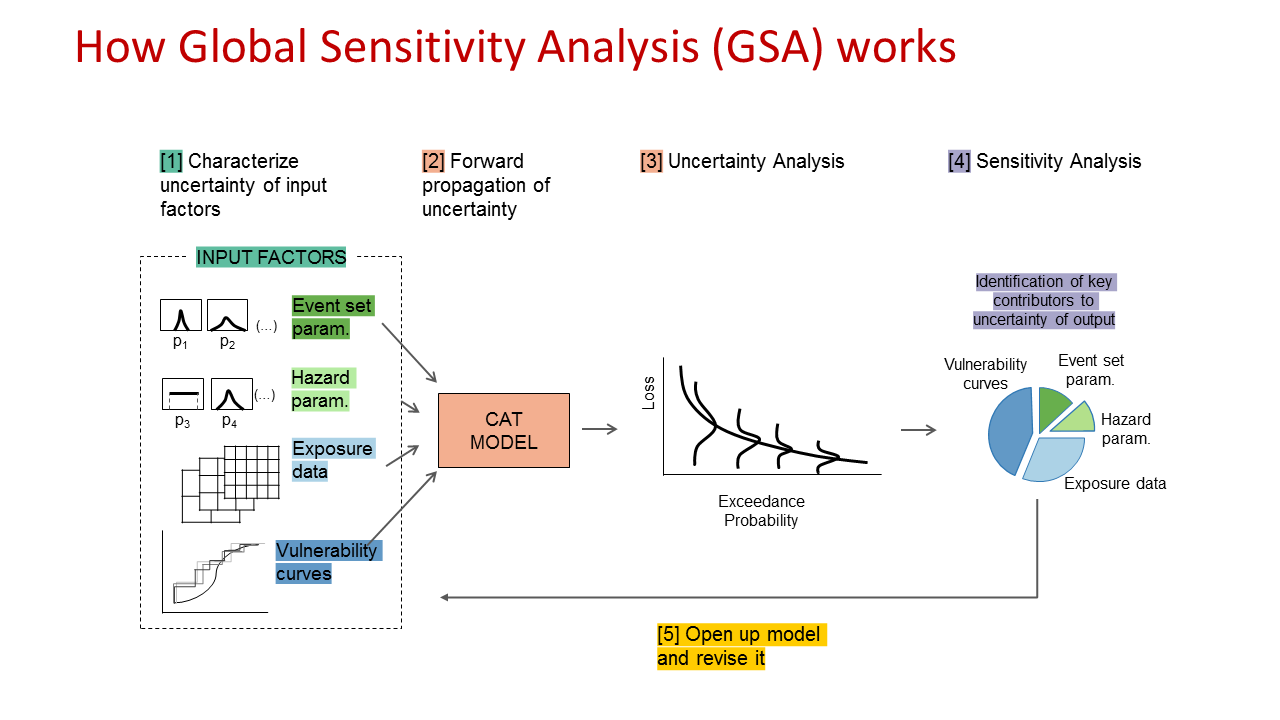## How to choose which GSA method to use?

It depends on the question you want SA to answer.

In general, GSA can have three purposes:

1. Ranking (or Factor Prioritization) to rank the input factors based on their relative contribution to the output variability.

2. Screening (or Factor Fixing) to identify the input factors, if any, which have a negligible influence on the output variability.

3. Mapping to determine the regions of the inputs’ variability space which produce output values of interest (e.g. extreme values).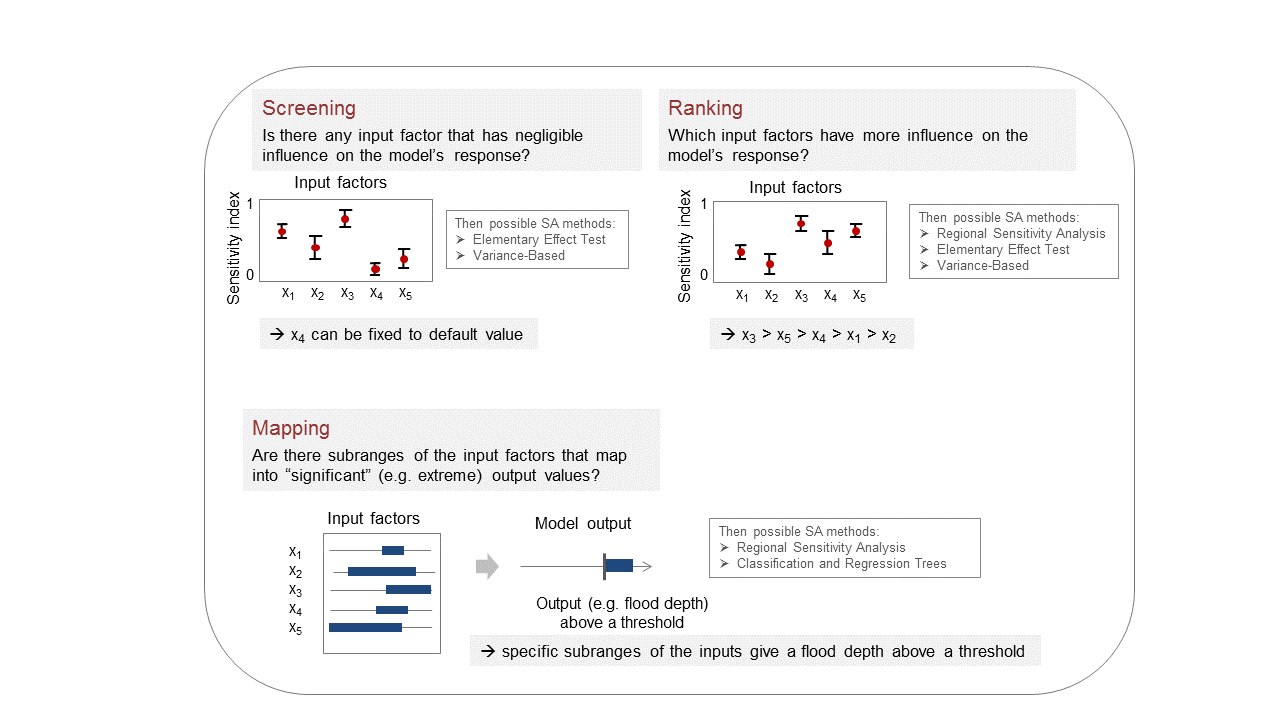## GSA workflow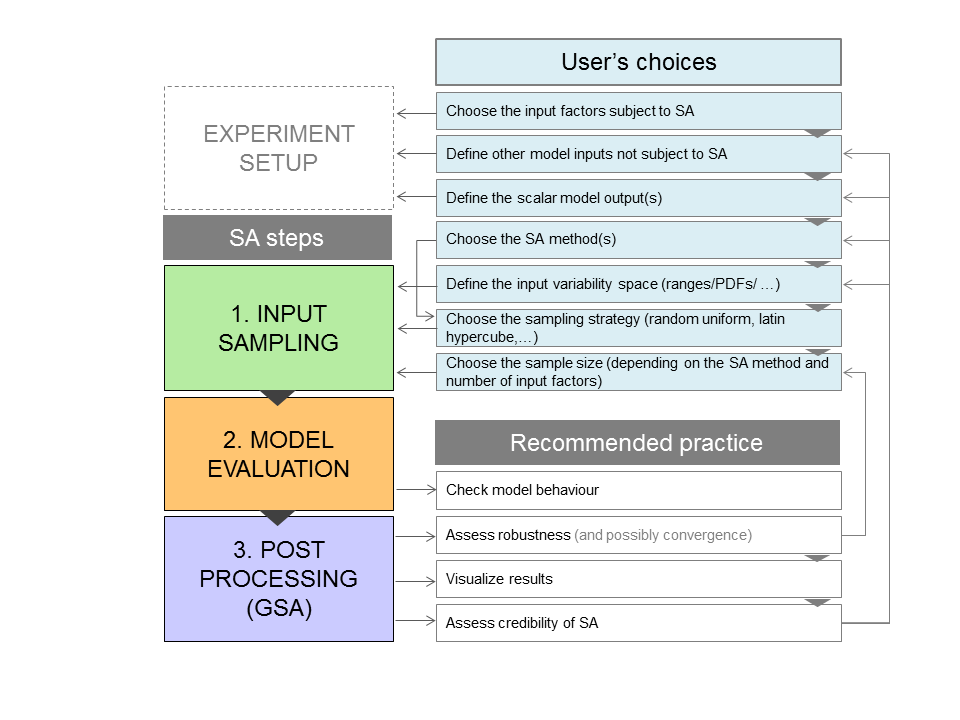There are 3 main steps in the GSA workflow:

1. Input Sampling

2. Model Execution

3. Post Processing (actual GSA routine)

But before starting there are a few choices one should take:

1. Choose the model input factors of which you want to investigate their influence on the model output through GSA.
2. Define the other model inputs not subject to GSA.
3. Define the scalar model output(s).

1. Afterwards, the Input Sampling requires the following steps:
1. Decide the GSA method(s) to use depending on the purpose of the analysis (e.g. ranking, screening, mapping).
2. Define the input variability space (i.e. the plausible range of values the inputs can take and their distribution).
3. Choose the sampling strategy (random uniform, Latin hypercube, …).
4. Choose the number of samples (depending on the GSA method and the number of input factors).

1. Run the model (Model Execution)

1. Post Processing (GSA)
1. Check that the model behaviour meets the expectations, and if not check whether this is due to any bug/error in the model.
2. Assess the robustness through bootstrapping to assess if the number of samples used is sufficient (and preferably the convergence of the sensitivity indices too).
3. Visualise the results (through scatter plots, parallel coordinate plots, …)
4. Assess the credibility of the GSA results (e.g. Is the impact of the varying inputs on the output as expected? Are the most influential inputs those expected? Is there any odd/unexpected behaviour? Are the confidence intervals of the sensitivity indices adequate for your purpose?)

Possibly use more than one GSA method to verify the consistency of the results.

### Step 1: Load the packages

Install and load the packages below.

library(caTools)
library(calibrater) # Install from tar file, also available at: https://people.maths.bris.ac.uk/~mazjcr/calibrater_0.51.tar.gz
library(SAFER) # Install from zip
library(ggplot2)
library(ggridges)
library(tidyr)
library(cowplot)

The Input Sampling step can be done either in R or in another environment, in the latter case go to Step 4b.

### Step 2: Define input factors subject to GSA

The choice of the distribution of the inputs DistrFun and their range DistrIn can be made by expert judgement, available data or literature sources.

In this case study the inputs are:

• vulnerability function (different number of steps are considered, discrete variable). For the purpose of this work basic vulnerability curves were chosen, but the model supports vulnerability curves of any level of detail deemed appropriate.

• buffer size (radius [m] of a circular buffer from which a flood depth is pulled from the flood map)

• number of disaggregation points (number of points into which aggregate exposure data is disaggregated)

DistrFun  <- c("unifd","unif","unif") # Inputs distribution
DistrIn  <- list( c(1, 3), c(0, 500), c(10000, 1000000))# Range of each input
x_labels <- c("Vuln. Func.","Buffer (m)","Disagg. Points") # Name of inputs

### Step 3: Sample inputs space

The number of model runs (N) typically increases proportionally to the number of input factors (M) and will depend on the GSA method chosen too. As a rule of thumb, it may require more than 10 model runs per input factor (M) for the most frugal methods (e.g. Elementary Effect Test) and more than 1000 model runs per M for the more expensive methods (e.g. Variance-Based) (see References 4-5 for further details).

SampStrategy <- "lhs" # Here the sampling strategy for All At the Time (AAT) sampling is
# Latin hypercube (another option is random uniform)
N <- 100 # Sample size
M <- length(DistrIn) # Number of inputs
X <- AAT_sampling(SampStrategy, M, DistrFun, DistrIn, N) # Sample inputs space
colnames(X) <- x_labels # Set columns names

### Step 4: Run the model

#### a) If the model is in R

Y <- flood_model(X) # Where 'flood_model' is your chosen model

#### b) If the model is in another environment

Run the model. Then load the file with the simulated inputs (one row per simulation and one column per input sampled) and with the simulated outputs (one row per simulation and one column per simulated output).

M <- 3 # Define number of input factors (if the model was run in another environment)
head(X) # Display first rows of the input factors to check format
##      Vuln_Func  Buffer Disagg_Points
## [1,]         3 258.014        659555
## [2,]         1 202.681        820321
## [3,]         1 308.082        508404
## [4,]         3 425.427        985957
## [5,]         1 474.902         81609
## [6,]         1  40.421        476490
n_out <- 14
head(Y) # Display first rows of the outputs to check format
##      aa_rp_1000 aa_rp_0750 aa_rp_0500 aa_rp_0250 aa_rp_0200 aa_rp_0100
## [1,]   41919.58   41066.08   39579.35   36098.45   35055.26   32662.33
## [2,]   38544.85   38170.91   36433.69   33878.36   32968.86   30717.68
## [3,]   38506.50   38003.53   36372.48   33844.15   33014.25   30753.27
## [4,]   41210.14   40458.26   38926.76   35817.45   34730.13   32380.68
## [5,]   38260.79   37938.46   36187.80   33752.56   32961.44   30590.38
## [6,]   39915.86   39194.48   37617.85   34172.07   33233.85   30626.22
##      aa_rp_0075 aa_rp_0050 aa_rp_0020 aa_rp_0015 aa_rp_0010 aa_rp_0005
## [1,]   31350.15   29013.31   18503.23   14567.20   11785.18    7787.64
## [2,]   29388.26   27229.60   17167.70   13563.14   11101.60    7333.06
## [3,]   29436.89   27171.85   17008.96   13417.24   10886.15    7133.89
## [4,]   31085.26   28722.11   18105.76   14410.89   11593.66    7579.62
## [5,]   29288.19   26996.41   16993.84   13340.02   10767.81    6981.15
## [6,]   29536.30   27243.80   17400.29   13968.97   11446.57    7649.14
##      aa_rp_0002     aal
## [1,]    2817.30 5088.08
## [2,]    2660.77 4784.17
## [3,]    2553.78 4683.38
## [4,]    2711.53 4967.26
## [5,]    2475.83 4602.65
## [6,]    2887.68 4989.25

### Step 5: Check model behaviour by visualising input/output samples

Use SAFER function scatter_plots to visualise inputs/outputs

x_labels <- c("Vuln. Func.","Buffer","Disagg. Points") # Name of inputs
sz_tx <- 12 # Font size for plots

N <- length(Y) # Get number of samples
colnames(X) <- x_labels # Set column names
p1 <- scatter_plots(X, Y[,14], prnam = x_labels) + ylab("AAL") +
theme(text = element_text(size=sz_tx)) + theme(axis.title.x=element_blank())
p2 <- scatter_plots(X, Y[,13], prnam = x_labels) + ylab("2-year Loss")  +
theme(text = element_text(size=sz_tx)) + theme(axis.title.x=element_blank())
p3 <- scatter_plots(X, Y[,5], prnam = x_labels) + ylab("200-yr Loss")  +
theme(text = element_text(size=sz_tx)) + theme(axis.title.x=element_blank())
plot_grid(p1, p2, p3, nrow = 3)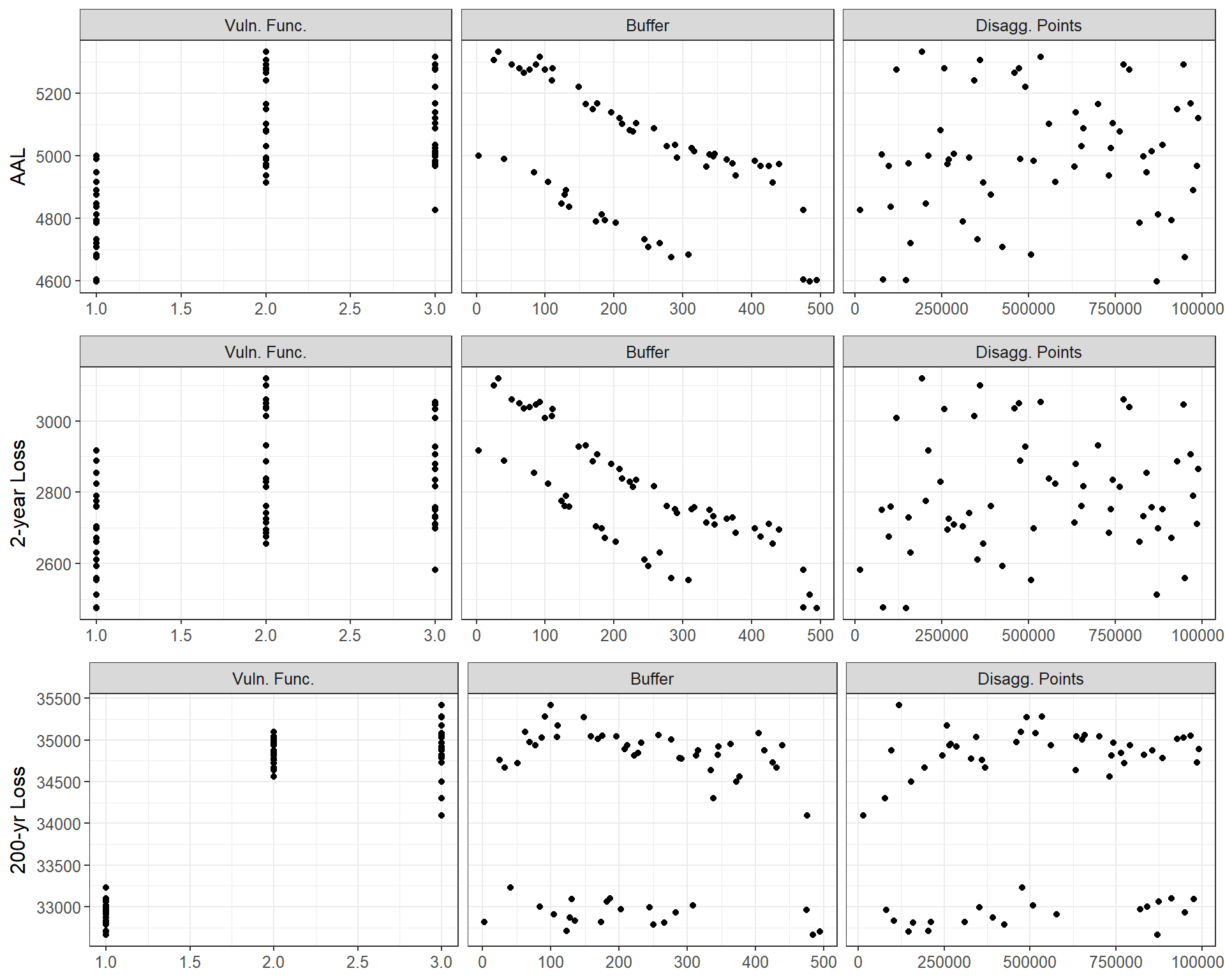Question: From these scatter plots, which input factor would you say is most influential? Why?
Are there input factors that are not influential at all? Does this depend on the output considered?

$\begin{array}{l} -------------------------------------------------------------- \\ \\ -------------------------------------------------------------- \\ \\ -------------------------------------------------------------- \\ \end{array}$

### Step 6: Uncertainty Analysis: Visualise outputs’ distributions

Visualise the distribution of the losses for different return periods.

Yu <-  read.csv("Yn.csv", header = T, fileEncoding="UTF-8-BOM")
Yu2 <- gather(Yu[, -ncol(Yu)])
ggplot(Yu2, mapping = aes(x = value, y = key)) + geom_density_ridges(scale = 5, size = 0.25, rel_min_height = 0.03, alpha = 0.7) + theme_ridges() +  xlab("Loss") + ylab("Return Period")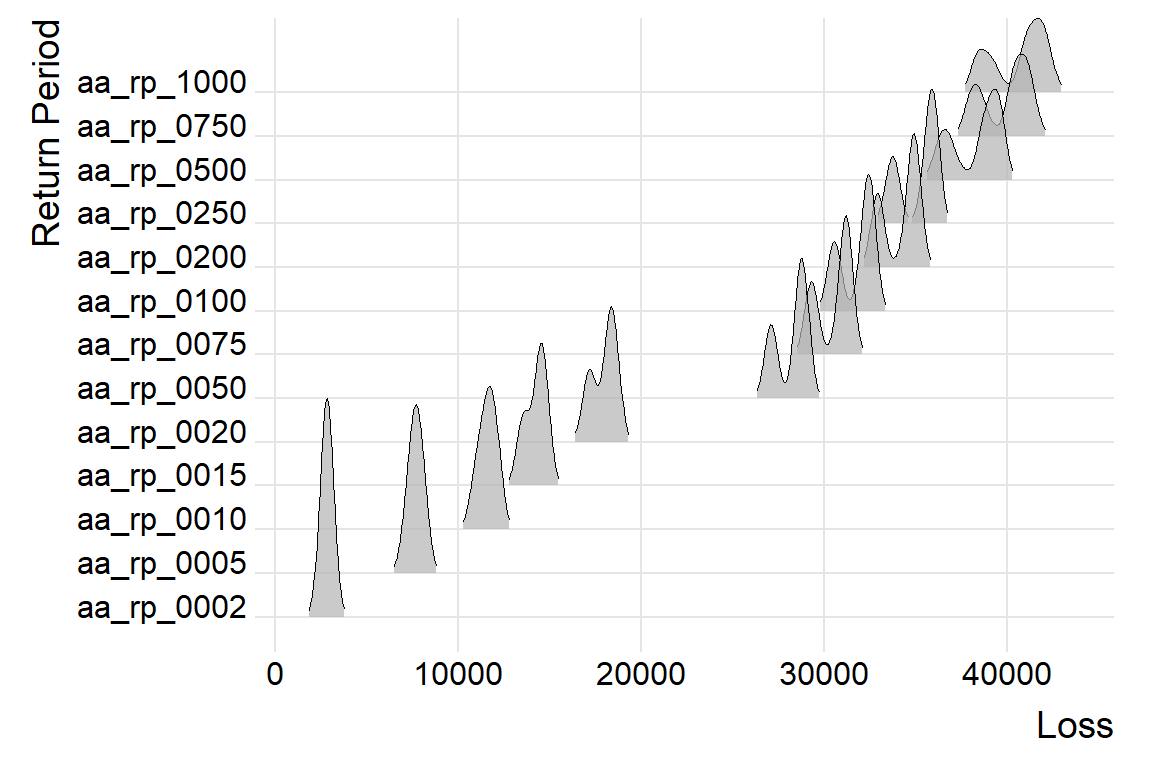### Step 7: Compute sensitivity indices with RSA

Let’s now apply Global Sensitivity Analysis: for example Regional Sensitivity Analysis (RSA), which aims at identifying regions in the inputs space corresponding to particular regions (e.g. high or low) of the output.

RSA requires to sort the output and then to split the output into different groups. Afterwards, we identify regions in the inputs space which produced the output in each group.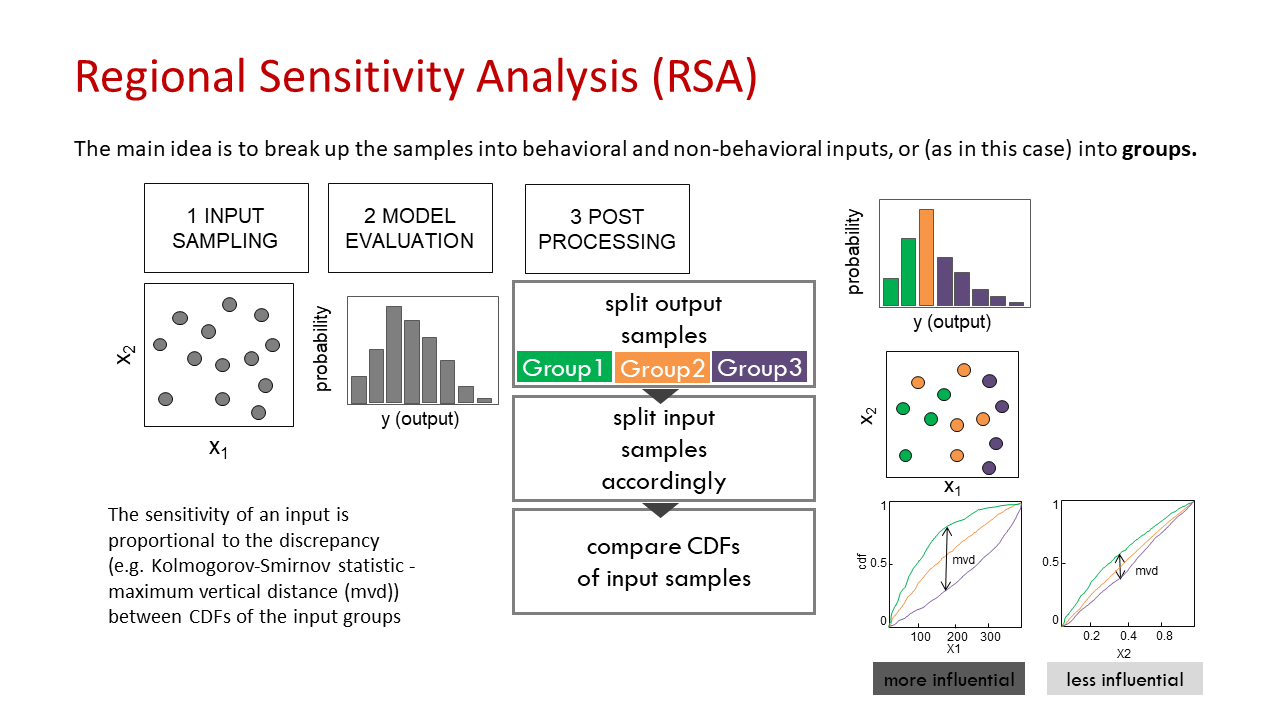So let’s divide the outputs into n_groups number of groups, where each group contains the same number of samples.

n_groups <- 5; # Number of groups into which the output is splitted, default = 10

flag <- 1; # where flag: statistic for the definition of the RSA index (scalar)
# flag <- 1: stat = median (default)
# flag <- 2: stat = maximum

rsa_gr_AAL <- RSA_indices_groups(X, Y[,14], n_groups, flag)

# Outputs
mvd_AAL <- rsa_gr_AAL$stat # mvd: maximum vertical distance between CDFs (sensitivity index) idx_AAL <- rsa_gr_AAL$idx # idx: index which divides the output into different groups
Yk_AAL <- rsa_gr_AAL$Yk # Yk: output limit of each group rsa_gr_2yr <- RSA_indices_groups(X, Y[,13], n_groups, flag) # Outputs mvd_2yr <- rsa_gr_2yr$stat # mvd: maximum vertical distance between CDFs (sensitivity index)
idx_2yr <- rsa_gr_2yr$idx # idx: index which divides the output into different groups Yk_2yr <- rsa_gr_2yr$Yk # Yk: output limit of each group

rsa_gr_200yr <- RSA_indices_groups(X, Y[,5], n_groups, flag)

# Outputs
mvd_200yr <- rsa_gr_200yr$stat # mvd: maximum vertical distance between CDFs (sensitivity index) idx_200yr <- rsa_gr_200yr$idx # idx: index which divides the output into different groups
Yk_200yr <- rsa_gr_200yr\$Yk # Yk: output limit of each group

### Step 8: Visualise input/output samples divided by group

Let’s now replot the results with the function scatter_plots where each group of inputs corresponds to a range of output (as estimated in Step 7) and is plotted with a different colour.

p1 <-scatter_plots(X, Y[,14], ngr = n_groups, prnam = x_labels) + ylab("AAL") +
theme(text = element_text(size=sz_tx)) + theme(axis.title.x=element_blank())
p2 <- scatter_plots(X, Y[,13], ngr = n_groups, prnam = x_labels) + ylab("2-year Loss") +
theme(text = element_text(size=sz_tx)) + theme(axis.title.x=element_blank())
p3 <- scatter_plots(X, Y[,5], ngr = n_groups, prnam = x_labels) + ylab("200-year Loss") +
theme(text = element_text(size=sz_tx)) + theme(axis.title.x=element_blank())
plot_grid(p1, p2, p3, nrow = 3)